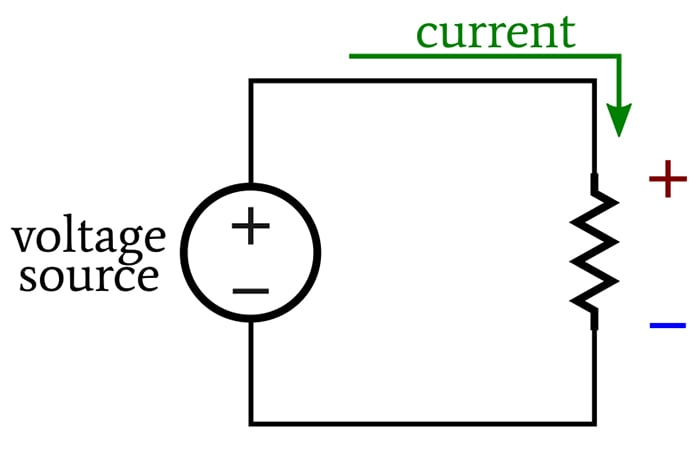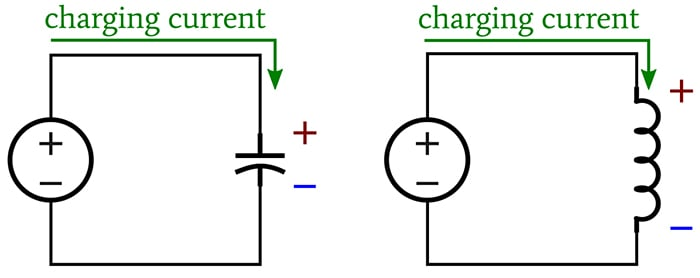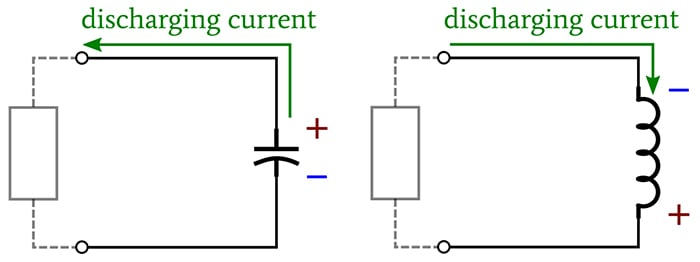Technical Article

# What Is a Voltage Drop in an Electric Circuit?

July 20, 2020 by Robert Keim

## How exactly does polarity factor into the voltage drops across the three basic elements of electrical circuits—resistors, capacitors, and inductors?

In this Frequent Engineering Question, we'll give a brief conceptual explanation of voltage drops and discuss the polarity of voltages dropped across resistors, capacitors, and inductors.

### Voltage, Work, and Current Flow

A battery converts chemical energy into electrical energy, producing a voltage—i.e., a difference in electric potential—across its two terminals. A resistor is a component that creates a specified amount of resistance to electric current. When we connect the two terminals of a resistor to the two terminals of a battery, charge carriers move through the circuit, and we call this electric current.

Voltage conveys the ability to do the work of moving charge from one point to another. A 5 V battery, for example, can do 5 joules of work per coulomb of charge. When current is flowing through a resistor, we can measure the amount of work (per unit charge) required to keep the current flowing through the resistor.

This is the essence of voltage drop: a battery (or voltage source) supplies energy for doing the work of moving charge. When current is flowing, components such as resistors consume energy, and the amount of work per unit charge associated with the current flowing through a given component is the component’s voltage drop.

The voltage dropped by a component accounts for a portion of the voltage generated by the battery. In other words, the work performed by the battery is divided up among the components in the circuit.

We can intuitively recognize that driving a given amount of current through more resistance will require more work. Thus, if two resistors are in series (meaning that they have the same current flow), the resistor with more resistance has a larger voltage drop. This is the basis for the operation of the voltage divider circuit.

### Polarity of Voltage Drops

A resistor always functions as a load—that is, as a component that consumes energy. If we adopt the conventional current flow model, in which current flows from higher voltage to lower voltage, the voltage drop across a resistor is positive where the current enters the resistor and negative where the current exits the resistor:##### A current flow model depicting how a voltage drop is positive where current enters a resistor and negative where it exits.

This polarity “opposes” the source voltage: if we connected a battery with this same polarity orientation, it would drive current in the opposite direction (or it would counteract the source voltage, depending on how you think about it).

Capacitors and inductors store energy, and consequently, they can function as either a load or a source. When they are acting as loads, they have the same voltage-drop polarity as a resistor.##### When acting as loads, capacitors and inductors have the same voltage-drop polarity as a resistor.

The voltage-drop polarity of a capacitor doesn’t change when it begins to discharge. Even though it is acting as a source, it produces current whose direction is opposite to that of the charging current.

However, when an inductor discharges, it attempts to maintain current flow. Thus, the polarity of the inductor’s voltage drop changes, because it is generating current whose direction is the same as that of the charging current produced by the source.##### Depiction of how an inductor attempts to maintain current flow when it discharges.

• Share• S
Slinger42 July 24, 2020

Would it be appropriate to emphasise that the driving force or EMF (measured in Volts) produced by the battery or source is achieved by, in the case of the battery, chemical reactions that effectively pile negative charges (electrons) on one plate by removing them from the other leaving behind a positive charge. When a load is connected the natural drive is for the electrons to travel around the circuit and get back to the positive plate to rebalance the situation. This drive is in fact the E.M.F. In flowing through the ‘load’ the electrons are depleted from the negative electrode but are replaced by the chemical reaction by electrons from the positive plate, and in doing so the current has to flow through the internal chemical constituents of the battery which also has a resistance to the flow. So the circuit shown above should have a source resistance showing, allowing one to estimate the new terminal voltage at the battery terminals which will now have fallen by an amount dependant on the source resistance. As the internal chemical constituents gradually degrade the internal resistance rises until the terminal voltage falls to such an extent that we consider the battery as flat.

Like.
• V
vanderghast July 24, 2020
True. Consider too that a plate (electrode) will pile negative charges while the other will be left with a deficit of electrons, and, placed between the two plates, the "load" will consume the energy. But the piling of electrons (and of "holes") in the plates will soon become higher than the emf and thus the current will soon stop. That is why there is a need to counter that effect by supplying another path. That other path cannot be a copper wire, as example, since that wire will become an easier path for the electrons which will then pass by that new wire instead of by the "load". That is a reason why the first batteries were with liquid! The electrons in the electrodes have great difficulties to get OUT of the solid metal, so they will mostly pass through the "load", while the liquid, with an appropriate amount of ions will allow "counter ions" ( + ions for the plate with an excess of electrons, and - ions for the plate with a lack of electrons ) will migrate in the liquid toward the plate which attract them and doing so, will neutralize the electrical potential built-up of the plate and the chemical reaction of redox (one metal is "reduced", the other is "oxyded", so the name of the chemical reaction, red(uction)-ox(ydation), or redox for short)) would then be able to continue. At least that is for electrolytic cells. So, there is a current, outside, through the conductive wire, and, inside the cell, through the liquid with ton of ions. The outside current is driven by the chemical redox reaction generating a voltage, while the internal current is driven by compensation of the electrons which have moved away/into the plates.
Like.
•MrChips July 28, 2020

While the expression “voltage drop” is commonly used when analyzing electronic circuits, it has led to much confusion to someone learning about circuits. It often leads to questions such as
“Why did the voltage drop?”
“What is the voltage drop at the base of the transistor?”

It would be best to avoid the use of the term “voltage drop” in introductory levels of electrical & electronics instruction.

The preferred expression is “potential difference”, such as, there is a potential difference between point-A and point-B. In other words, voltage does not drop. It doesn’t go away anywhere in the circuit. It is always there. It just depends on how and where you measure it.

Here is a hypothetical example. Connect a 0-ohm load across a 12V battery. What is the “voltage drop” across the load? Did it suddenly vanish to 0V?
Instead, we can experimentally measure the potential difference across the terminals of the battery and the terminals of the load. Then we can make theoretical assumptions about any surprises in observed measurements.

Like.
•Bill Collis July 28, 2020
There has been much research done on students misunderstandings in electric circuit theory. Students make significant errors with voltage as you state. We also see that students struggle with 'current'. I prefer to use electric potential and charge flow or flow of charge to help students begin to clarify what the commonly misused words of voltage and current mean.
Like.